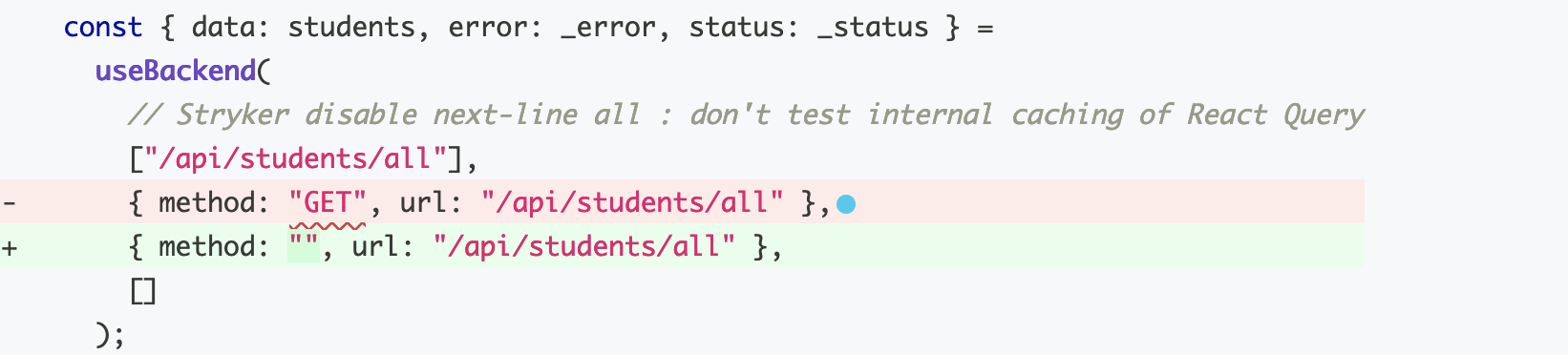Sometimes there is code that, when a mutation is applied, the resulting code will still pass the tests.

Here is an example (taken from the Strkyer documentationfor disabling mutations):

``````function max(a, b) {
return a < b ? b : a;
}
``````

One mutation that is applied here, the `EqualityOperator` mutation,
turns this into:

``````function max(a, b) {
return a <= b ? b : a;
}
``````

The thing is, that is still a correct solution, so this mutation will not be killed by any correct test.

We can fix this by using a comment to disable mutations. This comment disable all mutations on the next line of code:

``````function max(a, b) {
// Stryker disable next-line all
return a <= b ? b : a;
}
``````

But a better approach is to just disable the single mutation that is causing the trouble, since other mutations of this line still result in mutations that can and should be killed by tests:

``````function max(a, b) {
// Stryker disable next-line EqualityOperator
return a <= b ? b : a;
}
``````

It is possible to add an explanation to comments like this by putting a colon at the end, like this:

``````function max(a, b) {
// Stryker disable next-line EqualityOperator: The <= mutant results in an equivalent mutant
return a <= b ? b : a;
}
``````

Here is an example where `"GET"` is the default. Replacing it with empty string `""` results in an equivalent mutation: